# How to Calculate and Solve for Bouguer Anomaly | Gravity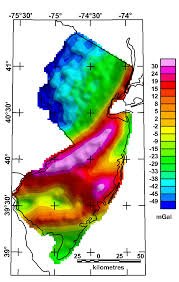The image above represents bourger anomaly.

To compute for bourger anomaly, three essential parameters are needed and these parameters are Free Air Correction (gF), Bouguer Correction (δgB) and Terrain Correction (δgT).

The formula for calculating bourger anomaly:

gB = gF – δgB + δgT

Where:

gB = Bouguer Anomaly
gF = Free Air Correction
δgB = Bouguer Correction
δgT = Terrain Correction

Let’s solve an example;
Find the bourger anomaly when the free air correction is 14, the bourger anomaly is 18 and the terrian correction is 20.

This implies that;

gF = Free Air Correction = 14
δgB = Bouguer Correction = 18
δgT = Terrain Correction = 20

gB = gF – δgB + δgT
gB = 14 – 18 + 20
gB = 14 – 38
gB = -24

Therefore, the bourger anomaly is -24 mGal.

Calculating the Terrian Correction when the Bourger Anomaly, the Free Air Correction and the Bourger Correction is Given.

δgT = gB – gF + δgB

Where;

δgT = Terrain Correction
gB = Bouguer Anomaly
gF = Free Air Correction
δgB = Bouguer Correction

Let’s solve an example;
Find the terrian correction when the bourger anomaly is 48, the free air correction is 22 and the bourger correction is 14.

This implies that;

gB = Bouguer Anomaly = 48
gF = Free Air Correction = 22
δgB = Bouguer Correction = 14

δgT = gB – gF + δgB
δgT = 48 – 22 + 14
δgT = 26 + 14
δgT = 40

Therefore, the terrian correction is 40.

Nickzom Calculator – The Calculator Encyclopedia is capable of calculating the bourger anomaly.

To get the answer and workings of the bourger anomaly using the Nickzom Calculator – The Calculator Encyclopedia. First, you need to obtain the app.

You can get this app via any of these means:

To get access to the professional version via web, you need to register and subscribe for NGN 2,000 per annum to have utter access to all functionalities.
You can also try the demo version via https://www.nickzom.org/calculator

Apple (Paid) – https://itunes.apple.com/us/app/nickzom-calculator/id1331162702?mt=8
Once, you have obtained the calculator encyclopedia app, proceed to the Calculator Map, then click on Geology under Add-on.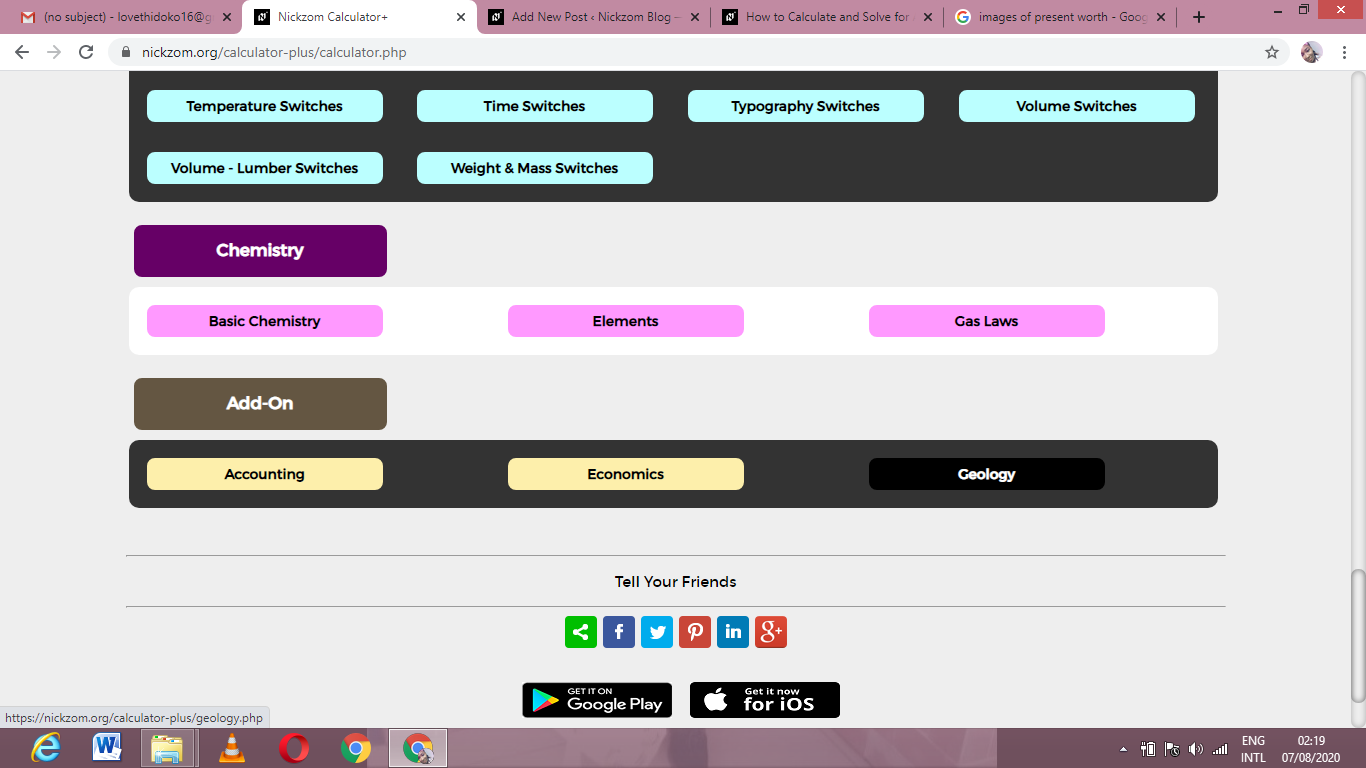Now, Click on Gravity under Geology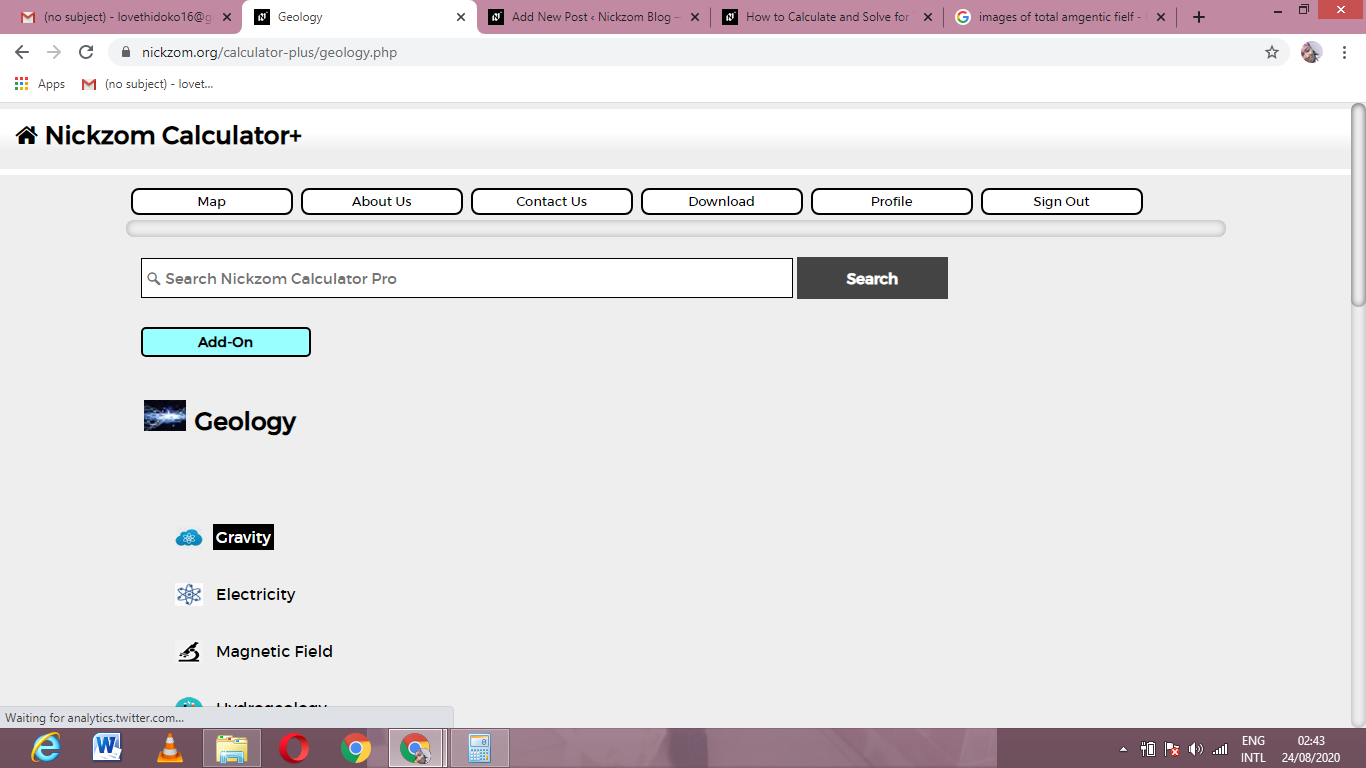Now, Click on Bourger Anomaly under Gravity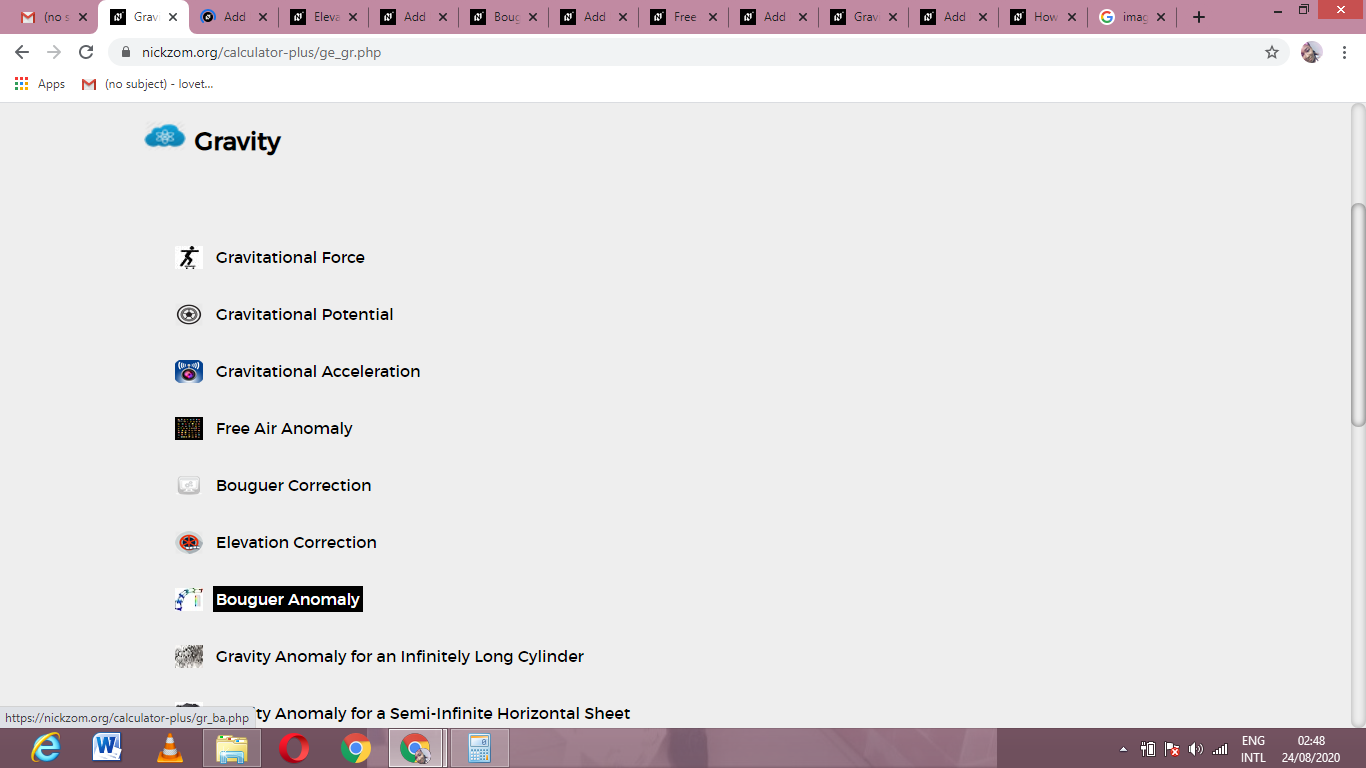The screenshot below displays the page or activity to enter your values, to get the answer for the bourger anomaly according to the respective parameters which are the Free Air Correction (gF), Bouguer Correction (δgB) and Terrain Correction (δgT).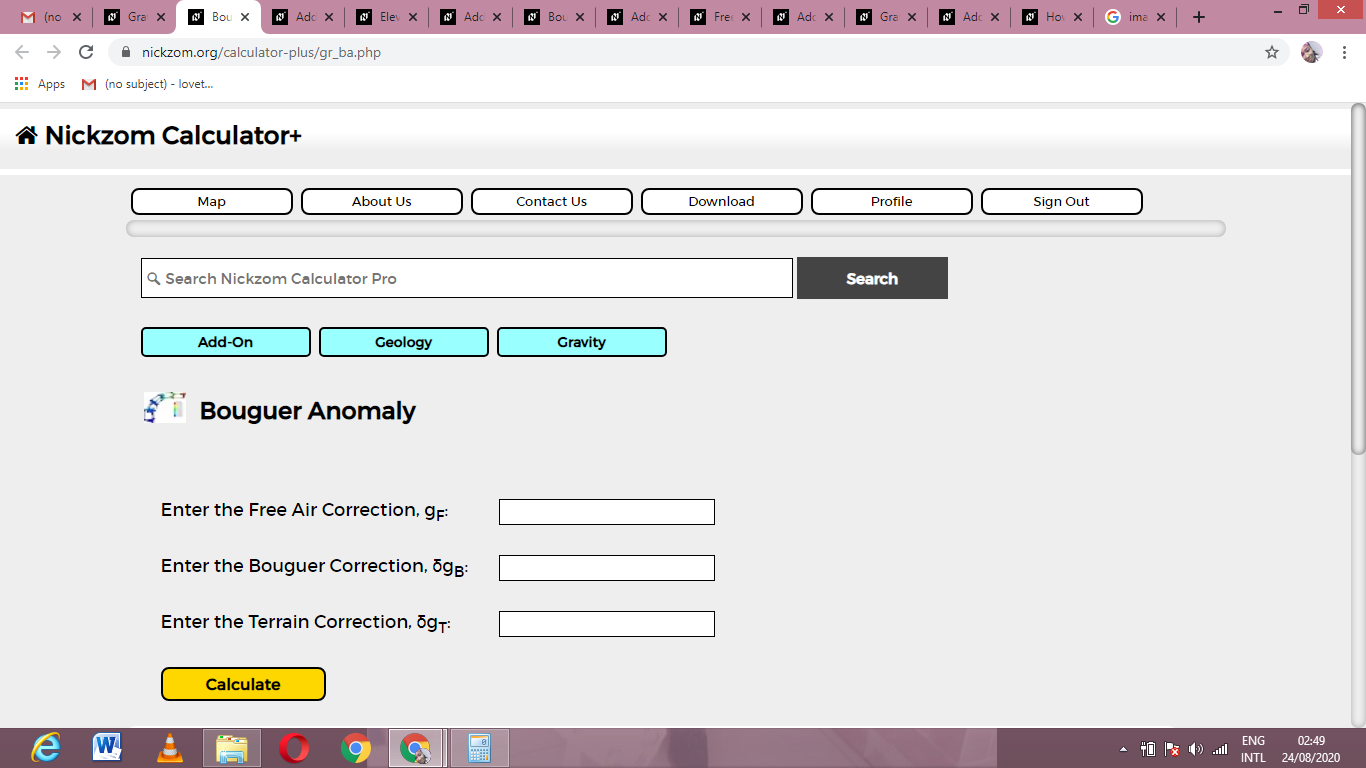Now, enter the values appropriately and accordingly for the parameters as required by the Free Air Correction (gF) is 14, Bouguer Correction (δgB) is 18 and Terrain Correction (δgT) is 20.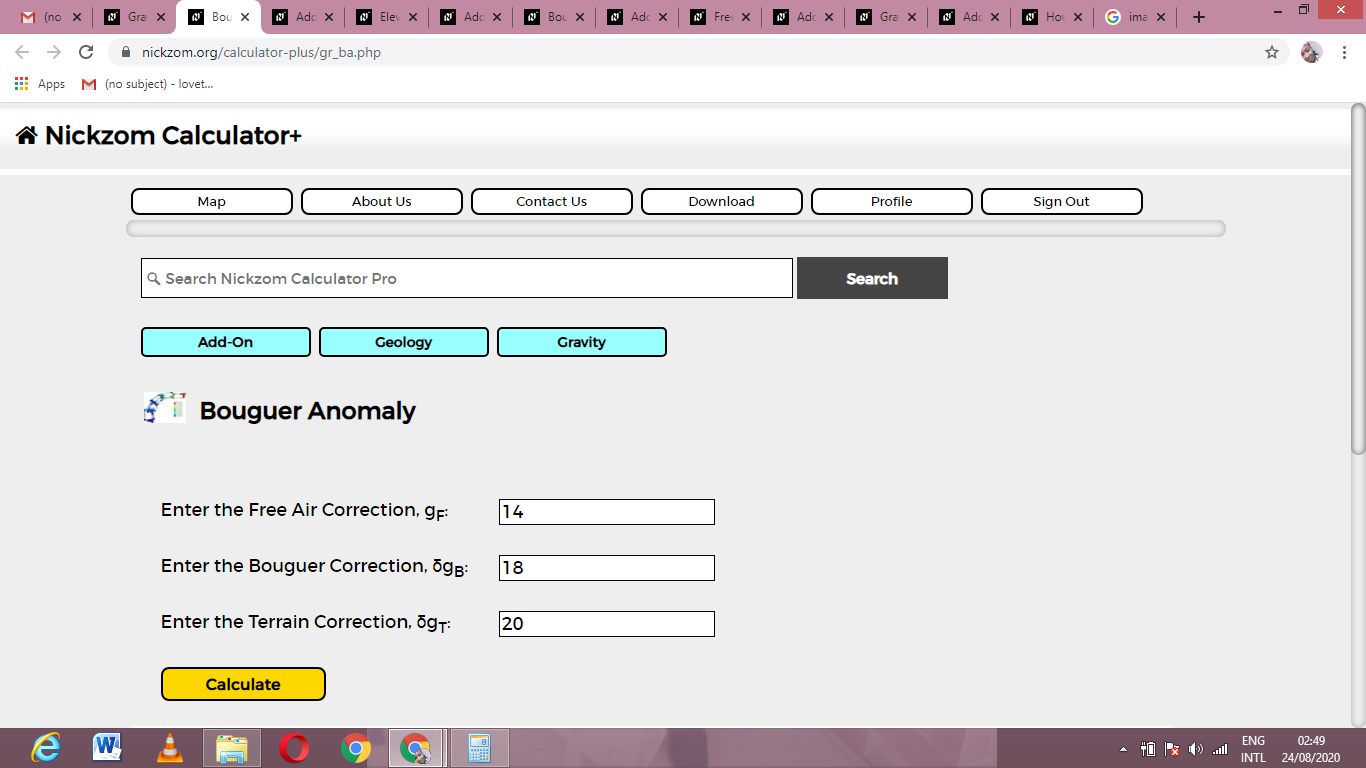Finally, Click on Calculate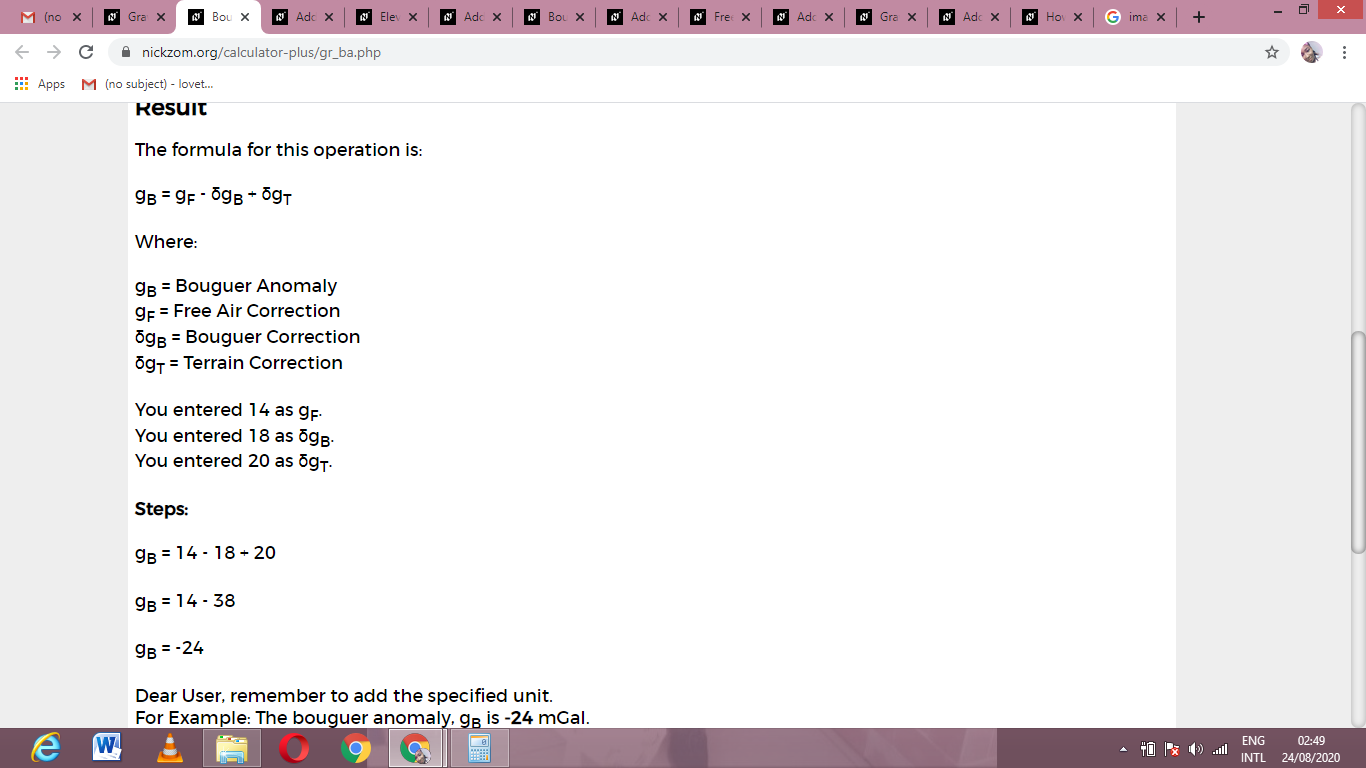As you can see from the screenshot above, Nickzom Calculator– The Calculator Encyclopedia solves for the bourger anomaly and presents the formula, workings and steps too.